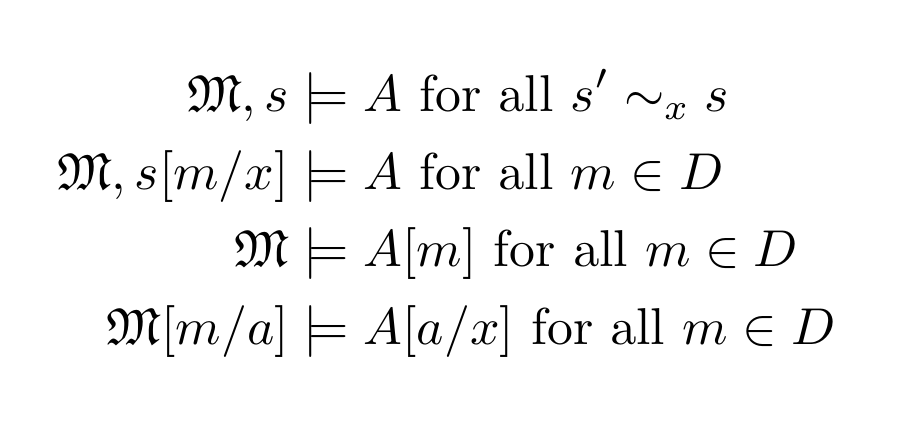# Satisfaction and assignments

When you define satisfaction for quantified formulas, e.g., $$\forall x\, A$$, you have to have a way to make $$x$$ range over all elements of the domain. Here are the common options: A. Tarski-style: use variable assignments $$s\colon V \to D$$ (where $$V$$ is the set of variables and $$D$$ the domain), then define \[\mathfrak{M}, … Continue reading Satisfaction and assignments# Letter grades in Brightspace/D2L (or other LMS)

So, we're all moving to online courses, and for some of us that means we have to figure out how to switch from scribbling feedback and letter grades on papers, handing them back to students, and turning those letter grades into a course grade at the end. Most of us are using learning management systems … Continue reading Letter grades in Brightspace/D2L (or other LMS)